3. General Theorems: Regular and Semi-Regular Tilings

This is section 3 of document Constructing Semi-Regular Tilings which is based on a talk given at the Spring 1995 Meeting of the Seaway Section of the Mathematical Association of America. The meeting was held at Hobart and William Smith Collleges in Geneva, New York on April 21--22, 1995.

The complete document is divided into five sections:

This section begins with restatements of some well-known results concerning regular and semi-regular tilings. Subsequently, a description of a process that allows one to "construct" semi-regular tilings from regular ones is given. Various vertex types are explicitly constructed and illustrated using tilings from the hyperbolic plane.

Theorem 1 (Regular Tilings). There is a regular tiling of type p^q for all p, q >= 3. In particular,

1. the regular tilings of the Euclidean plane are: 3^6, 4^4, and 6^3;
2. the regular tilings of the sphere (Platonic solids) are: 3^3, 3^4, 3^5, 4^3, and 5^3;
3. the regular tilings of the hyperbolic plane are: p^q where 1/ p + 1/q < 1/2.

Discussion of Theorem 1: The regular tilings of the sphere and Euclidean plane can be constructed by hand. The existence of the regular tilings of the hyperbolic plane is a bit more difficult to prove. One can piece together a proof from references in [C-M], [Mag], and [Ca]. In what follows, I will assume that these tilings exist.

Theorem 2 (Kepler). There are 8 semi-regular tilings of the Euclidean plane: 3.12^2, 4.6.12, 4.8^2, 3.4.6.4, 3.6.3.6, 3^4.6, 3^3.4^2, and 3^2.4.3.4.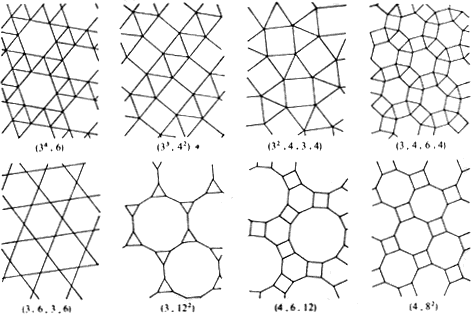Theorem 3 (Archimedes). The semi-regular tilings of the sphere are:
• the Archimedean solids: 3.6^2, 4.6^2, 5.6^2, 3.8^2, 3.10^2, 3.4.3.4, 3.5.3.5, 3.4^3, 3^4.4, 3^4.5, 4.6.8, 4.6.10, and 3.4.5.4;
• the prisms: 4^2.m, for m = 3 or m > 4,
• the anti-prisms: 3^3.n, for n > 3.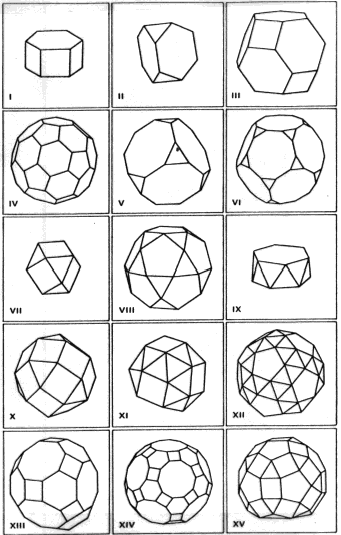1. 4^2.6 (prism with m = 6)
2. 3.6^2
3. 4.6^2
4. 5.6^2
5. 3.8^2
6. 3.10^2
7. 3.4.3.4
8. 3.5.3.5
9. 3^3.6 (anti-prism with m = 6)
10. 3.4^3
11. 3^4.4
12. 3^4.5
13. 4.6.8
14. 4.6.10
15. 3.4.5.4

Addtional illustrations of these tilings may be found in [G-V, Figure 31], [FT, Figure 17/2] and [P].

Discussion of Theorems 2 and 3: To construct the semi-regular tilings, the typical method is to proceed in two steps:

1. eliminate all vertex types except those enumerated;
2. actually construct the tilings listed.

The elimination process, uses the fact that the measure of a vertex angle of a regular p-gon is

• equal to Pi - (2*Pi/p) in the Euclidean plane;

• greater than Pi - (2*Pi/p) on the sphere;

• less than Pi - (2*Pi/p) in the hyperbolic plane.

Because the angles at any vertex of a tiling sum to 2*Pi, p_1.p_2 . . . p_q is a semi-regular tiling only if (after a bit of simplification)

• in the Euclidean plane: 1/p_1 + 1/p_2 + . . . + 1/p_q = (q-2)/2;

• on the sphere: 1/p_1 + 1/p_2 + . . . + 1/p_q > (q-2)/2;

• in the hyperbolic plane: 1/p_1 + 1/p_2 + . . . + 1/p_q < (q-2)/2.

In addition to the vertex types given by Kepler, the following types satisfy the first equation above but cannot be extended beyond a small patch of tiles to give a full tiling of the Euclidean plane: 3.7.42, 3.8.24, 3.9.18, 3.10.15, 4.5.20, 5^2.10, 3^2.6^2, 3^2.4.12, 3.4.3.12, and 3.4^2.6.

Similarly the following vertex types satisfy the second equation above but do not lead to tilings of the sphere: 3^2.n (n > 3), 5^2.n (n = 3,4,6,7,8,9), 3.7^2, 3.9^2, 3^2.4.n (4 <= n <=11), 3^2.5.n (5 <= n <= 7), 3.4^2.5, 3.4.3.n (5 <= n <=11), 3.5.3.n (n = 6,7).

Elimination By Parity

Lemma 1 (Parity Lemma). Let T be a semi-regular tiling.

1. Assume that p is odd and that to within reflection the adjacent pair of tiles x.p occurs in the vertex type of T only as part of the triple of consecutive tiles x.p.y and the pair p.y occurs in T only as part of the triple x.p.y. Then x = y.

2. Assume that p is odd and occurs in T (within reflection) only as part of four consecutive tiles of the form x.p.p.y. Then either x = p or y = p.

Proof: The hypotheses of (1) imply that the polygons x and y must alternate around consecutive sides of every p-gon that is adjacent to either an x-gon or a y-gon. But this is impossible since p is odd. The proof of (2) is similar.

A check shows that (1) eliminates all the potential vertex types listed above with the exception of 3^2.4.12 which is eliminated by (2).

For further parity results relating to vertex types of semi-regular tilings, see [Z] or [W].

Existence of Derived Tilings

Theorem 4. If the regular tiling p^q exists, then the following regular and semi-regular tilings also exist:
1. the dual regular tiling q^p;
2. p.2q.2q and 2p.2p.q;
3. 4.2p.2q;
4. p.q.p.q;
5. 4.p.4.q;
6. 3^2.p.3.q;
7. 3.p.3.p.3.(q/2), for q >= 6 and q even.

This result implies the existence of all of Kepler's and Archimedes' semi-regular tilings, except for the prisms, the anti-prisms, and the 3^3.4^2 tiling of the Euclidean plane. But all of these are easily constructed by hand.

Constructions

Theorem 4 is, itself, a consequence of a much more general result.

Theorem 5 (The Incenter Process). Given a (possibly non-regular) q-gon such that:

1. there is a tiling of the plane using only this k-gon in which adjacent tiles are reflections of each other across their shared edge;
2. the k-gon has an incenter.
Then the incenters of adjacent tiles may be joined to produce a semi-regular tiling p_1.p_2 . . . p_q, where p_i is the number of tiles at the i-th vertex of the q-gon.

Proof: The first condition implies that all the angles at a single vertex are reflections of each other. If p_i tiles meet at the i-th vertex, then the angle between consecutive edges there is 2*Pi/p_i, where 1 <= i <= q.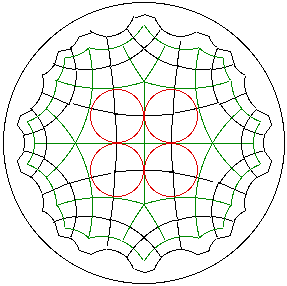If we connect the incenters of the adjacent polygons about the i-th vertex, a p_i-gon is formed. Every edge of this p_i-gon is composed of two collinear congruent radii of the incircles of adjacent q-gons. So the p_i-gon is equilateral. Since the vertex angles of the p_i-gon are also congruent (being reflected one into the next across adjacent edges of the q-gon) the p_i-gon is actually regular. Thus, at each incenter of the original tiling we have constructed a sequence of regular polygons p_1.p_2 . . . p_q. The sequence is the same at every incenter, since adjacent in q-gons are reflected onto each other across shared edges. So we have constructed the desired tiling.

Example. A reflection tiling (green) of the hyperbolic plane using 'kites' with angles Pi/6, Pi/2, 2*Pi/5, and Pi/2. These kites have circles inscribed in them (red). The centers of these circles are the vertices of a semi-regular 6.4.5.4 tiling (black).

Proof of Theorem 4: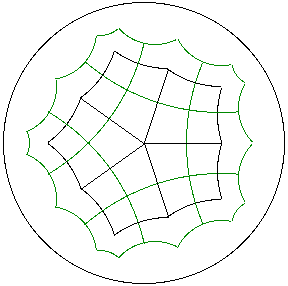1. Existence of the Dual q^p.
Since a regular p-gon has an incenter and since there are q tiles at all p vertices of the p-gon, the p^q tiling satisfies the hypotheses of Theorem 5. This proves that the dual regular tiling q^p exists.

Example. The regular 5^4 tiling of the hyperbolic plane in black and its dual regular tiling p^q = 4^5 in green. The vertices of the dual tiling lie at the center of the original 5-gons.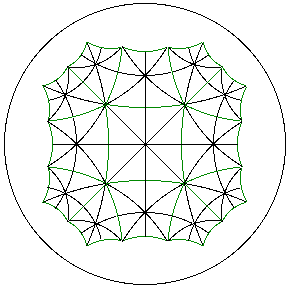2. Existence of p.2q.2q.
Divide each tile of p^q into p congruent isosceles triangles by connecting the center of the p-gon to each of its vertices. Since every triangle has an incenter, this produces a triangle tiling of the plane. Since reflections across all edges of these triangles are, in fact, symmetries of the original p^q, they are also symmetries of the triangle tiling. Thus, the triangle tiling satisfies the conditions of Theorem 5. Since the summit angle of the triangle (at the center of the p-gon) is 2*Pi/p and the base angles are Pi/q = 2*Pi/2q, we obtain a p.2q.2q. Applying this result to the dual q^p tiling produces a 2p.2p.q.

Example. A triangulation, in black, of the p^q = 4^5 tiling, in green, of the hyperbolic plane. The isosceles triangles have angles Pi/4, Pi/4, and Pi/5. Note that all triangles have incenters.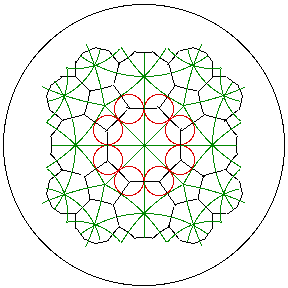The triangles, now in green, have inscirbed circles, here in red. Connecting centers of these circles produces the 2p.2p.q = 8.8.5 semi-regular tiling of the hyperbolic plane, in black. Note that the triangles are reflected one into the other across their edges and that all of these reflections are symmetries of the original 4^5.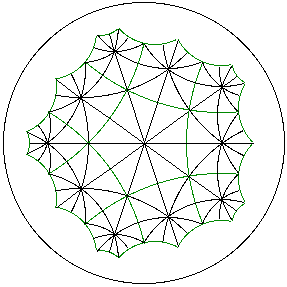3. Existence of 4.2p.2q.
Subdivide each p-gon of the original tiling into 2p triangles by connecting the center of the p-gon to each of its vertices and to the midpoint of each of its edges. The triangles within a p-gon are transformed successively into each other by reflections across the edges emanating from the center of the p-gon. Using reflections across the original edges of the p-gon allows us to transform the triangles of any one p-gon into any other. Thus the conditions of Theorem 5 are satisfied. Since the angles of the triangle are Pi/2, Pi/p, and Pi/q, we obtain a 4.2p.2q.

Example. Starting with the p^q = 5^4 tiling of the hyperbolic plane, in green, we form a triangulation, consisting of all the black and green edges, by right triangles with angles Pi/2, Pi/5, and Pi/4. The vertices of triangles are the centers and vertices of the original p-gons as well as the midpoints of each of the edges.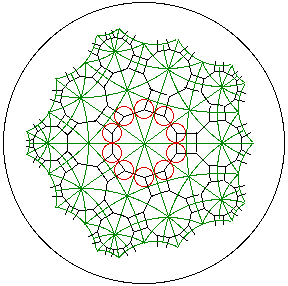But all triangles, now in green, have inscirbed circles (in red). Connecting centers of these circles produces the 4.2p.2q = 4.10.8 semi-regular tiling of the hyperbolic plane, here in black.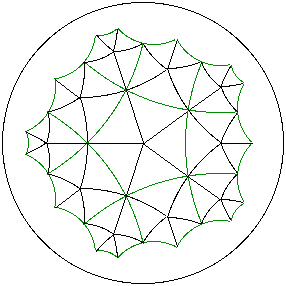4. Existence of p.q.p.q.
Form a tiling by rhombi by using only the segments from the centers of the p^q to the vertices of the p^q. Adjacent rhombi are reflections of each other across edges they share. Each rhombus has an incenter at the intersection of its diagonals. Since the angles of the rhombus are alternately 2*Pi/p and 2*Pi/q, we obtain a p.q.p.q.

Example. The green p^q = 5^4 tiling of the hyperbolic plane and its associated tiling by rhombii. Each rhombus has angles 2*Pi/5, Pi/2, 2*Pi/5, and Pi/2).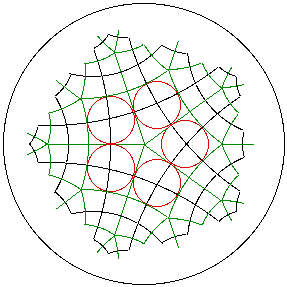Every green rhombus has an incircle (red) with center at the intersection of its diagonals. Connecting these centers produces the p.q.p.q = 5.4.5.4 semi-regular tiling (black) of the hyperbolic plane.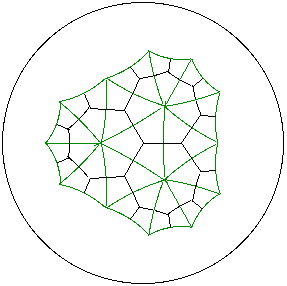5. Existence of 4.p.4.q.
If we overlay the dual q^p tiling on the p^q tiling, a tiling by kites with angles PI/2, 2*PI/p, PI/2, and 2*PI/q is created. Each kite has an incenter (at the intersection of its four angle bisectors) and so we obtain a 4.p.4.q.

Example. A p^q = 3^7 regular tiling, in green, of the hyperbolic plane with its dual q^p = 7^3 tiling, in black. Together these tilings form 'kites' with with angles Pi/2, 2*Pi/3, Pi/2 and 2*pi/7.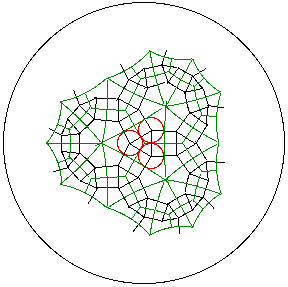Every kite (in green) has an incircle (red) with center at the intersection of its angle bisectors. Connecting these centers creates the 4.p.4.q = 4.3.4.7 semi-regular tiling (black) of the hyperbolic plane.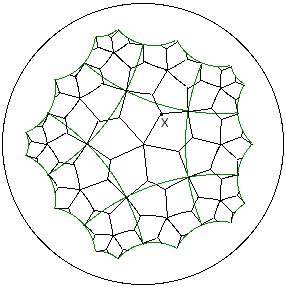6. Existence of 3.3.p.3.q.
Form irregular pentagons with angle 2*PI/p at the center of the p-gon, 2*PI/3 at the 'appropriate' interior point X of the p^q, 2*PI/q at a vertex of the p^q, and then consecutive angles of 2*PI/3 at the image of X under a rotation of 2*PI/q about the vertex and the image of X under a rotation of -2*PI/p about the center. Note: These pentagons are not reflected into each other across their shared edges. Rather, the rotations of 2*Pi/p about the center of each p-gon of the original p^q and the rotations of 2*p/q about each vertex of the p-gons are symmetries of this pentagonal tiling. Thus, the pentagonal tiling is 'sufficiently symmetric' for a modified version of Theorem 5 to apply.

Example. A tiling by irregular pentagons (in black) with angles 2*Pi/3, 2*Pi/3, 2*Pi/5, 2*Pi/3, Pi/2, and 2*Pi/3. Rotational symmetries of the underlying p^q = 5^4 regular tiling (green) are used to tile the plane with these pentagons.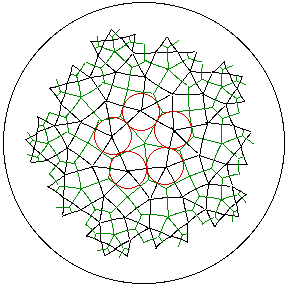Each pentagon (in green) has an incircle (in red). Connecting centers of these creates the 3^2.p.3.q = 3^2.5.3.4 semi-regular tiling of the hyperbolic plane, here in black.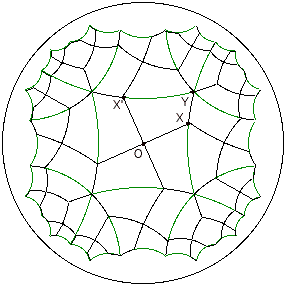7. Existence of 3.p.3.p.3.q/2.
Form irregular hexagons using a vertex Y of the p^q, an appropriate point X on an edge of the p^q containing Y, the center of the p-gon O, the image X' of X under a rotation of 2*PI/p about O. The remainder of the hexagon is obtained by reflecting across the edge containing Y and X'. Symmetries of this hexagonal tiling include rotations of 2*Pi/p about the centers of the original p-gons and reflections across the edges of the p-gons. The proof of Theorem 5 can be adapted to this situation.

Example. A tiling by irregular hexagons (in black) with angles 2*Pi/3, Pi/3, 2*Pi/3, Pi/2, 2*Pi/3, and 2*Pi/5 based on the underlying p^q = 4^6 tiling (in green) of the hyperbolic plane. The hexagon tiling can be rotated about the center of any 4-gon and reflected across the edge of any 4-gon.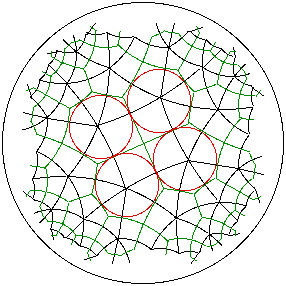Each hexagon (in green) has an incircle (red). Connecting centers of these creates the 3.p.3.p.3.q/2 = 3.4.3.4.3.3 semi-regular tiling of the hyperbolic plane, here in black.

Using Theorem 4 and the existence of the regular tilings of the Euclidean plane and the sphere in Theorem 1, we obtain most of the semi-regular tilings in the Euclidean plane and on the sphere listed in Theorems 2 and 3. These are shown in the table below, along with some reperesentative hyperbolic tilngs.

Regular  Dual  p.2q^2  4.2p.2q  p.q.p.q  4.p.4.q  3^2.p.3.q  3.p.3.p.3.q/2

Euclidean   3^6	  6^3  3.12^2   4.6.12  3.6.3.6  4.3.4.6      3^5.6  3^6
4^4	  4^4   4.8^2    4.8^2      4^4      4^4    3^4.3.4
6^3	  3^6     6^3   4.6.12  3.6.3.6  4.3.4.6      3^5.6

Sphere      3^3   3^3   3.6^2    4.6^2      3^4  3.4.3.4        3^5
3^4   4^3   3.8^2    4.6.8  3.4.3.4    3.4^3      3^4.4
3^5   5^3  3.10^2   4.6.10  3.5.3.5  3.4.5.4      3^4.5
4^3   3^4   3.8^2    4.6.8  3.4.3.4    3.4^3      3^4.4
5^3   3^5  3.10^2   4.6.10  3.5.3.5  3.4.5.4      3^4.5

Hyperbolic  3^7   7^3  3.14^2   4.6.14  3.7.3.7  3.4.7.4      3^5.7
4^5   5^4  4.10^2   4.8.10  4.5.4.5    4^3.5  3^2.4.3.5
4^6   6^4  4.12^2   4.8.12  4.6.4.6    4^3.6  3^2.4.3.6  3^3.4.3.4
5^8   8^5  5.16^2  4.10.16  5.8.5.8  4.5.4.8  3^2.5.3.8  3.4.3.5.3.5
To complete the proof of Theorems 2 and 3, note that the remaining 5-valent semi-regular tiling in the Euclidean plane is 3^3.4^2. It is easily constructed by hand using alternating parallel bands of equilateral triangles and squares. Similarly, the prisms 4^2.n on the sphere are constructed by using a band of n 4-gons capped above and below by an n-gon and the antiprisms 3^3.n are formed by taking a band of 2*n equilateral triangles and again capping with n-gons.

Though we have not outlined how to produce the regular tilings in Theorem 1, using (1) of Theorem 4, we see that in the Euclidean plane the hexagon tiling 6^3 is dual to the triangle tiling 3^6 and on the sphere the octahedron 3^4 is dual to the cube 4^3 and the dodecahedron 5^3 is dual to the icosahedron 3^5. So we can get away with constructing the square tiling 4^4 and triangle tiling 3^6 in the Euclidean plane and the tetrahedron 3^3, cube 4^3, and icosahedron 3^5 on the sphere.

Of course, Theorems 4 and 5 also apply to the hyperbolic plane. For a further discussion see 4. Hyperbolic Results. For additional sources of material, see the References section.

Author: Kevin Mitchell (mitchell@hws.edu)
Last Update: 13 February 1996.